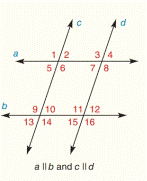Chapter 2.CR, Problem 3CRElementary Geometry For College St...

7th Edition
Alexander + 2 others
ISBN: 9781337614085

Solutions

Chapter
SectionElementary Geometry For College St...

7th Edition
Alexander + 2 others
ISBN: 9781337614085
Textbook Problem

Given: m ∠ 9 = 2 x + 17 m ∠ 11 = 5 x − 94 Find: xTo determine

To find:

The value of x.

Explanation

Given:

The given statement is,

m9=2x+17m11=5x94abcd

Figure (1)

Property:

If two parallel lines are cut by a transversal, then a pair of corresponding angles is congruent.

Calculation:

The given statement is,

m9=2x+17m11=5x94abcd

9 and 11 are corresponding angles

Still sussing out bartleby?

Check out a sample textbook solution.

See a sample solution

The Solution to Your Study Problems

Bartleby provides explanations to thousands of textbook problems written by our experts, many with advanced degrees!

Get Started

A population of N = 15 scores has SX = 120. What is the population mean?

Statistics for The Behavioral Sciences (MindTap Course List)

In Exercises 7-10, find the slope of the line shown in each figure. 7.

Applied Calculus for the Managerial, Life, and Social Sciences: A Brief Approach

In Problems 35-38, use the x-intercept method to find one solution of each equation.

Mathematical Applications for the Management, Life, and Social Sciences